# quantum mechanics

Also found in: Dictionary, Thesaurus, Medical, Acronyms, Wikipedia.
Related to quantum mechanics: String theory, theory of relativity, Quantum numbers

## quantum mechanics:

see quantum theoryquantum theory,
modern physical theory concerned with the emission and absorption of energy by matter and with the motion of material particles; the quantum theory and the theory of relativity together form the theoretical basis of modern physics.
.

## Quantum mechanics

The modern theory of matter, of electromagnetic radiation, and of the interaction between matter and radiation; also, the mechanics of phenomena to which this theory may be applied. Quantum mechanics, also termed wave mechanics, generalizes and supersedes the older classical mechanics and Maxwell's electromagnetic theory. Atomic and subatomic phenomena provide the most striking evidence for the correctness of quantum mechanics and best illustrate the differences between quantum mechanics and the older classical physical theories. Quantum mechanics is needed to explain many properties of bulk matter, for instance, the temperature dependence of the specific heats of solids.

The formalism of quantum mechanics is not the same in all domains of applicability. In approximate order of increasing conceptual difficulty, mathematical complexity, and likelihood of future fundamental revision, these domains are the following: (i) Nonrelativistic quantum mechanics, applicable to systems in which particles are neither created nor destroyed, and in which the particles are moving slowly compared to the velocity of light. Here a particle is defined as a material entity having mass, whose internal structure either does not change or is irrelevant to the description of the system. (ii) Relativistic quantum mechanics, applicable in practice to a single relativistic particle (one whose speed equals or nearly equals c); here the particle may have zero rest mass, in which event, its speed must equal c. (iii) Quantum field theory, applicable to systems in which particle creation and destruction can occur; the particles may have zero or nonzero rest mass. This article is concerned mainly with nonrelativistic quantum mechanics, which apparently applies to all atomic and molecular phenomena, with the exception of the finer details of atomic spectra. Nonrelativistic quantum mechanics also is well established in the realm of low-energy nuclear physics. See Atomic structure and spectra, Nuclear physics, Quantum field theory, Relativistic quantum theory

#### Planck's constant

The quantity 6.626 × 10-34 joule-second, first introduced into physical theory by Max Planck in 1901, is a basic ingredient of the formalism of quantum mechanics. Planck's constant commonly is denoted by the letter h; the notation &hstrok; = h/2π also is standard.

#### Uncertainty principle

In classical physics the observables characterizing a given system are assumed to be simultaneously measurable (in principle) with arbitrarily small error. For instance, it is thought possible to observe the initial position and velocity of a particle and therewith, using Newton's laws, to predict exactly its future path in any assigned force field. According to the uncertainty principle, accurate measurement of an observable quantity necessarily produces uncertainties in one's knowledge of the values of other observables. In particular, for a single particle relation (1a)

(1a)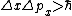(1b)holds, where Δx represents the uncertainty (error) in the location of the x coordinate of the particle at any instant, and Δpx is the simultaneous uncertainty in the x component of the particle momentum. Relation (1a) asserts that under the best circumstances, the product ΔxΔpx of the uncertainties cannot be less than about 10-34 joule second.

The uncertainty relation (1b) is derived and interpreted somewhat differently than relation (1a); it asserts that for any system, an energy measurement with error ΔE must be performed in a time not less than Δt ∼ &hstrok;/ΔE. If a system endures for only Δt seconds, any measurement of its energy must be uncertain by at least ΔE ∼ &hstrok;Δt. See Uncertainty principle

#### Wave-particle duality

It is natural to identify such fundamental constituents of matter as protons and electrons with the mass points or particles of classical mechanics. According to quantum mechanics, however, these particles, in fact all material systems, necessarily have wavelike properties. Conversely, the propagation of light, which, by Maxwell's electromagnetic theory, is understood to be a wave phenomenon, is associated in quantum mechanics with massless energetic and momentum-transporting particles called photons. The quantum-mechanical synthesis of wave and particle concepts is embodied in the de Broglie relations, given by Eqs. (2a) and (2b).

(2{\it a})(2{\it b})These give the wavelength λ and wave frequency f associated with a free particle (a particle moving freely under no forces) whose momentum is p and energy is E; the same relations give the photon momentum p and energy E associated with an electromagnetic wave in free space (that is, in a vacuum) whose wavelength is λ and frequency is f. See Photon

The wave properties of matter have been demonstrated conclusively for beams of electrons, neutrons, atoms (hydrogen, H, and helium, He), and molecules (H2). When incident upon crystals, these beams are reflected into certain directions, forming diffraction patterns. Diffraction patterns are difficult to explain on a particle picture; they are readily understood on a wave picture, in which wavelets scattered from regularly spaced atoms in the crystal lattice interfere constructively along certain directions only. See Electron diffraction, Neutron diffraction

The particle properties of light waves are observed in the photoelectric effect and the compton effect. See Compton effect, Photoemission

#### Complementarity

Wave-particle duality and the uncertainty principle are thought to be examples of the more profound principle of complementarity, first enunciated by Niels Bohr (1928). According to the principle of complementarity, nature has “complementary” aspects; an experiment which illuminates one of these aspects necessarily simultaneously obscures the complementary aspect. To put it differently, each experiment or sequence of experiments yields only a limited amount of information about the system under investigation; as this information is gained, other equally interesting information (which could have been obtained from another sequence of experiments) is lost. Of course, the experimenter does not forget the results of previous experiments, but at any instant, only a limited amount of information is usable for predicting the future course of the system.

#### Quantization

In classical physics the possible numerical values of each observable, meaning the possible results of exact measurement of the observable, generally form a continuous set. For example, the x coordinate of the position of a particle may have any value between -∞ and +∞. In quantum mechanics the possible numerical values of an observable need not form a continuous set, however. For some observables, the possible results of exact measurement form a discrete set; for other observables, the possible numerical values are partly discrete, partly continuous; for example, the total energy of an electron in the field of a proton may have any positive value between 0 and +∞, but may have only a discrete set of negative values, namely, -13.6, -13.6/4, -13.6/9, -13.6/16 eV,…. Such observables are said to be quantized; often there are simple quantization rules determining the quantum numbers which specify the allowable discrete values. Spectroscopy, especially the study of atomic spectra, probably provides the most detailed quantitative confirmation of quantization.

#### Probability considerations

The uncertainty and complementarity principles, which limit the experimenter's ability to describe a physical system, must limit equally the experimenter's ability to predict the results of measurement on that system. Suppose, for instance, that a very careful measurement determines that the x coordinate of a particle is precisely x = x0. This is permissible in nonrelativistic quantum mechanics. Then, formally, the particle is known to be in the eigenstate corresponding to the eigenvalue x = x0 of the x operator. Under these circumstances, an immediate repetition of the position measurement again will indicate that the particle lies at x = x0. Knowing that the particle lies at x = x0 makes the momentum px of the particle completely uncertain, however, according to relation (1a). A measurement of px immediately after the particle is located at x = x0 could yield any value of px from -∞ to +∞.

More generally, suppose the system is known to be in the eigenstate corresponding to the eigenvalue α of the observable A. Then for any observable B, which is to some extent complementary to A, that is, for which an uncertainty relation of the form of relations (1) limits the accuracy with which A and B can simultaneously be measured, it is not possible to predict which of the many possible values B = β will be observed. However, it is possible to predict the relative probabilities Pα(β) of immediately thereafter finding the observable B equal to β, that is, of finding the system in the eigenstate corresponding to the eigenvalue B = β.

To the eigenvalues correspond eigenfunctions, in terms of which Pα &lsim; β can be computed. In particular, when α is a discrete eigenvalue of A, and the operators depend only on x and px, the probability Pα(β) is postulated as in

(3)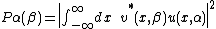Eq. (3), where u(x,α) is the eigenfunction corresponding to A = α; v(x,β) is the eigenfunction corresponding to B = β; and the * denotes the complex conjugate. The integral in Eq. (3) is called the projection of u(x,α) on u(x,β). The quantity &verbar;u(x,α)&verbar;2 dx is the probability that the system, known to be in the eigenstate A = α, will be found in the interval x to x + dx. See Eigenvalue (quantum mechanics)

#### Wave function

When the system is known to be in the eigenstate corresponding to A = α, the eigenfunction u(x,α) is the wave function; that is, it is the function whose projection on an eigenfunction v(x,β) of any observable B gives the probability of measuring B = β. The wave function ψ(x) may be known exactly; in other words, the state of the system may be known as exactly as possible (within the limitations of uncertainty and complementarity), even though ψ(x) is not the eigenfunction of a known operator. This circumstance arises because the wave function obeys Schrödinger's wave equation. Knowing the value of ψ(x) at time t = 0, the wave equation completely determines ψ(x) at all future times. In general, however, if ψ(x,0) = u(x,α), that is, if ψ(x,t) is an eigenfunction of A at t = 0, then ψ(x,t) will not be an eigenfunction of A at later times t > 0.

A system described by a wave function is said to be in a pure state. Not all systems are described by wave functions, however. For example, a beam of hydrogen atoms streaming out of a small hole in a hydrogen discharge tube can be regarded as a statistical ensemble or mixture of pure states oriented with equal probability in all directions.

#### Schrödinger equation

Equation (4) describes a plane wave

(4)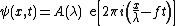of frequency f, wavelength λ, and amplitude A(λ), propagating in the positive x direction. The previous discussion concerning wave-particle duality suggests that this is the form of the wave function for a beam of free particles moving in the x direction with momentum p = px, with Eq. (2) specifying the connections between f, λ, and E, p. Differentiating Eq. (4), it is seen that Eqs. (5) hold. Since for a free particle E = p2/2m, it follows also that Eq. (6) is valid.
(5{\it a})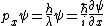(5{\it b})(6)See W ave motion

Equation (6) holds for a plane wave of arbitrary λ, and therefore for any superposition of waves of arbitrary λ, that is, arbitrary px. Consequently, Eq. (6) should be the wave equation obeyed by the wave function of any particle moving under no forces, whatever the projections of the wave function on the eigenfunctions of px. Equations (5) and (6) further suggest that for a particle whose potential energy V(x) changes, in other words, for a particle in a conservative force field, ψ(x,t) obeys Eq. (7).

(7)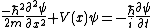Equation 7 is the time-dependent Schrödinger equation for a one-dimensional (along x), spinless particle. Noting Eq. (5b), and observing that Eq. (7) has a solution for the form of Eq. (8), it is inferred that ψ(x) of Eq. (8) obeys the time-dependent Schrödinger equation, Eq. (9).

(8)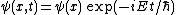(9)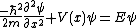See F orce

Equation (9) is solved subject to reasonable boundary conditions, for example, that ψ must be continuous and must not become infinite as x approaches ±∞. These boundary conditions restrict the values of E for which there exist acceptable solutions ψ(x) to Eq. (9), the allowed values of E depending on V(x). In this manner, the allowed energies of atomic hydrogen listed in the earlier discussion of quantization are obtained.

The forms of Eqs. (5a), (7), and (9) suggest that the classical observable px, must be replaced by the operator (&hstrok;/i) (∂/∂x). With this replacement, Eq. (10) holds.

(10)In other words, whereas the classical canonically conjugate variables x and px are numbers, obeying the commutative law in Eq. (11a), the quantum-mechanical quantities x and px are noncommuting operators, obeying Eq. (11b).
(11{\it a})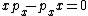(11{\it b})#### Correspondence principle

Since classical mechanics and Maxwell's electromagnetic theory accurately describe macroscopic phenomena, quantum mechanics must have a classical limit in which it is equivalent to the older classical theories. Although there is no rigorous proof of this principle for arbitrarily complicated quantum-mechanical systems, its validity is well established by numerous illustrations.

McGraw-Hill Concise Encyclopedia of Physics. © 2002 by The McGraw-Hill Companies, Inc.

## quantum mechanics

see quantum theory.
Collins Dictionary of Astronomy © Market House Books Ltd, 2006
The following article is from The Great Soviet Encyclopedia (1979). It might be outdated or ideologically biased.

## Quantum Mechanics

(wave mechanics), a theory that establishes the laws of motion of microparticles (elementary particles, atoms, molecules, atomic nuclei) and their systems (for example, crystals) and a method of describing their motions; it also establishes the relation between quantities characterizing particles and systems and the physical quantities directly measured in macroscopic experiments.

The laws of quantum mechanics form the foundation of the study of the structure of matter. They have made it possible to determine the structure of atoms, to establish the nature of the chemical bond, to explain the periodic table of the elements, to understand the structure of atomic nuclei, and to study the properties of elementary particles. Insofar as the properties of macroscopic bodies are determined by the motion and interaction of the particles of which they consist, the laws of quantum mechanics underlie an understanding of most macroscopic phenomena. Quantum mechanics has made it possible, for example, to explain the temperature dependence and calculate the magnitude of the specific heat of gases and solids and to determine the structure and understand many properties of solids (metals, dielectrics, semiconductors). Only on the basis of quantum mechanics has it been possible to explain consistently such phenomena as ferromagnetism, superfluidity, and superconductivity; to understand the nature of such astrophysical objects as white dwarfs and neutron stars; and to determine the mechanism of thermonuclear reactions in the sun and stars. There are also phenomena (such as the Josephson effect) in which the laws of quantum mechanics are directly manifested in the behavior of macroscopic objects.

A number of the most important technical achievements of the 20th century have been effectively based on specific laws of quantum mechanics. For example, quantum-mechanical laws underlie the operation of nuclear reactors and make thermonuclear reactions possible under terrestrial conditions; they are manifested in a number of phenomena in metals and semiconductors used in modern technology. The quantum-mechanical theory of radiation constitutes the foundation of such a rapidly developing branch of physics as quantum electronics. The laws of quantum mechanics are used in the search for and development of new materials (especially magnetic, semiconductor, and superconducting materials). Thus, quantum mechanics is becoming largely an “engineering” science a knowledge of which is necessary not only for research physicists but also for engineers.

Place among other sciences of motion. Early in the 20th century it became evident that the classical mechanics of I. Newton had a limited range of application and required generalization. First, it is inapplicable at high rates of motion of bodies—at velocities comparable to the velocity of light. Here it was replaced by relativistic mechanics, constructed on the basis of A. Einstein’s special theory of relativity. Relativistic mechanics includes Newtonian (nonrelativistic) mechanics as a particular case. Hereafter, the term “classical mechanics” will include Newtonian and relativistic mechanics.

The description of particles by specifying their positions in space (their coordinates) and their velocities and the dependence of these quantities on time is characteristic of classical mechanics as a whole. The motion of particles along well-defined trajectories corresponds to such a description. However, experiment has shown that this description is not always valid, especially for particles of very small mass (microparticles). This fact constitutes a second limitation on the applicability of Newtonian mechanics. A more general description of motion is given by quantum mechanics, which includes classical mechanics as a particular case. Quantum mechanics, like classical mechanics, is divided into nonrelativistic mechanics, which is valid in the case of low velocities, and relativistic mechanics, which satisfies the requirements of the special theory of relativity. The foundations of nonrelativistic quantum mechanics are presented in this article. (However, some general propositions apply to quantum mechanics as a whole.) Nonrelativistic quantum mechanics, like Newtonian mechanics for its range of applicability, is a fully complete and logically self-consistent theory that in principle is capable of giving, within its own sphere of competence, a quantitative solution to any physics problem. Relativistic quantum mechanics is not so complete and self-consistent. Whereas in the nonrelativistic domain it may be assumed that motion is determined by forces that act (instantaneously) at a distance, this is not true in the relativistic domain. Insofar as, according to the theory of relativity, interaction is transmitted (propagated) with finite velocity, there must exist a physical agent that transmits the interaction; the field is such an agent. The difficulties of relativistic theory are the difficulties of field theory, which are encountered by both relativistic classical mechanics and relativistic quantum mechanics. The problems of relativistic quantum mechanics connected with quantum field theory will not be considered in this article.

Criteria of applicability of classical mechanics. The relationship between Newtonian and relativistic mechanics is determined by the existence of a fundamental quantity, the maximum rate of signal propagation, which is equal to the velocity of light c(c ≈ 3 × 1010 cm/sec). If the velocity of a body v is much less than that of light (that is, v/c « 1, so that c may be considered to be infinitely large), then Newtonian mechanics is applicable.

The relationship between classical mechanics and quantum mechanics is less graphic in character. It is determined by the existence of another universal constant—Planck’s constant h. The constant h (also called the quantum of action) has the dimensions of action (energy multiplied by time) and is equal to h = 6.62 × 10−27 erg.sec. (The quantity ℏ = h/2π = 1.0545919 × 10−27 erg• sec—Dirac constant—is used more often in theory; it is also called the Planck constant.) The criterion of applicability of classical mechanics formally consists in the following: classical mechanics is applicable if under the conditions of a given problem the physical quantities with the dimension of action are much greater than ℏ (so that ℏ may be considered very small). This criterion will be explained in greater detail during the exposition of the physical principles of quantum mechanics.

History. Two groups of phenomena (seemingly unrelated) attesting to the inapplicability of conventional classical Newtonian mechanics and classical electromagnetic field theory (classical electrodynamics) to processes of the interaction of light with matter and to processes occurring within the atom were discovered in the early 20th century. The first group of phenomena was connected with the experimental establishment of the dual nature of light (the duality of light); the second was connected with the impossibility of explaining, on the basis of classical concepts, the stable existence of the atom and the spectral regularities discovered during the study of the emission of light by atoms. The establishment of a relationship between these groups of phenomena and the attempts to explain them on the basis of a new theory ultimately led to the discovery of the laws of quantum mechanics.

Quantum concepts (in particular, the quantum constant h) were first introduced into physics by M. Planck’s work (1900) on the theory of thermal radiation. The then-current theory of thermal radiation, constructed on the basis of classical electrodynamics and statistical physics, led to the meaningless result that thermal (thermodynamic) equilibrium between radiation and matter cannot be attained, since sooner or later all energy must go over into radiation. On the basis of an extraordinarily bold hypothesis, Planck resolved the contradiction and obtained results that agreed remarkably with experiment. In contrast to the classical theory of radiation, which considers the emission of electromagnetic waves as a continuous process, Planck assumed that light is emitted in definite bundles of energy—quanta. The magnitude of such a quantum of energy depends on the frequency of the light v and is equal to ε = hv.

Two interconnected lines of development that culminated in the final formulation of quantum mechanics in its two forms by 1927 can be traced from this work by Planck. The first begins with Einstein’s work (1905) in which the theory of the photoelectric effect—the phenomenon of the light-induced emission of electrons from matter—was given. In developing Planck’s idea, Einstein assumed that not only is light emitted and absorbed in discrete bundles—quanta of radiation—but light is also propagated in such quanta, that is, discreteness is inherent in light itself; light itself consists of separate bundles—quanta of light, which were later called photons. The energy £ of a photon is related to the wave’s oscillation frequency v by Planck’s formula ε = hv. On the basis of this hypothesis, Einstein explained the regularities of the photoelectric effect, which contradicted the classical theory of light (which is based on classical electrodynamics).

Further proof of the corpuscular nature of light was obtained in 1922 by A. Compton, who experimentally proved that the scattering of light by free electrons takes place according to the laws of elastic collisions between two particles—a photon and an electron. The kinematics of such a collision is determined by the laws of conservation of energy and momentum, and, in addition to the energy ε = hv, the momentum p = h/λ = hv/c, where λ is the length of the light wave, must be ascribed to the photon. The energy and momentum of a photon are related by the formula ε = cp, which is valid in relativistic mechanics for a particle with zero mass.

Thus, it was proved experimentally that in addition to known wave properties (which are manifested, for example, in the diffraction of light), light also has corpuscular properties: it consists, as it were, of particles—photons. The duality of light and its complex wave-particle nature are manifested herein. The duality is already present in the formula ε = hv, which does not make it possible to choose either of the two concepts: in the left part of the equality the energy ε applies to a particle, but in the right part, the frequency v is a characteristic of a wave. A formal logical contradiction arose: to explain certain phenomena it was necessary to assume that light has a wave nature, but to explain other phenomena, it must have a corpuscular nature. The resolution of this contradiction essentially led to the development of the physical foundations of quantum mechanics.

In 1924, L. de Broglie, seeking an explanation for the conditions postulated in 1913 by N. Bohr for the quantization of atomic orbits (see below ), advanced the hypothesis that wave-particle duality is universal. According to de Broglie, to every particle, regardless of its nature, there should correspond a wave whose length λ is related to the momentum of the particle p by the formula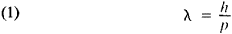According to this hypothesis, not only photons but all “ordinary particles” (such as electrons and protons) as well have wave properties that should, in particular, be manifested in the phenomenon of diffraction. In 1927, C. Davisson and L. Germer first observed the diffraction of electrons. Later, wave properties were also observed for other particles, and the validity of de Broglie’s formula was confirmed experimentally. In 1926, E. Schrödinger proposed an equation to describe the behavior of such “waves” in external force fields. Wave mechanics thus arose. Schrödinger’s wave equation is the fundamental equation of nonrelativistic quantum mechanics. In 1928, P. Dirac formulated a relativistic equation describing the motion of an electron in an external force field; Dirac’s equation has become one of the basic equations of relativistic quantum mechanics.

The second line of development begins with Einstein’s work (1907) on the theory of the specific heat of solids (which is also a generalization of Planck’s hypothesis). Electromagnetic radiation consisting of a set of electromagnetic waves of different frequencies is dynamically equivalent to some set of oscillators. The emission or absorption of the waves is equivalent to the excitation or damping of the corresponding oscillators. The fact that emission and absorption of electromagnetic radiation by matter take place in quanta of energy hv may be expressed in the following manner: an electromagnetic field oscillator cannot have arbitrary energy and can have only certain energy values —discrete energy levels, the distance between which is equal to hv. Einstein generalized this idea of the quantization of the energy of an electromagnetic field oscillator to an oscillator of arbitrary nature. Inasmuch as the thermal motion of solids reduces to the vibrations of atoms, a solid is dynamically equivalent to a set of oscillators. The energy of such oscillators is also quantized, that is, the difference between adjacent energy levels (the energies that the oscillator may have) must be equal to hv, where v is the vibration frequency of the atoms. Einstein’s theory, refined by P. Debye, M. Born, and T. von Kármán, played a prominent role in the development of solid-state theory.

In 1913, Bohr applied the idea of the quantization of energy to the theory of atomic structure, a planetary model of which followed from the results of E. Rutherford’s experiments (1911). According to this model, a positively charged nucleus, in which virtually the entire mass of the atom is concentrated, is located at the center of the atom; negatively charged electrons revolve about the nucleus in orbits. Examination of such motion on the basis of classical concepts led to the paradoxical result of the impossibility of the stable existence of atoms: according to classical electrodynamics, the electron cannot move in a stationary orbit since a revolving electric charge must radiate electromagnetic waves and consequently lose energy; the radius of its orbit must decrease and in a period of the order of 10−8 sec the electron should fall into the nucleus. This meant that the laws of classical physics were inapplicable to the motion of electrons in the atom, since atoms exist and are extremely stable.

To explain the stability of atoms, Bohr assumed that of all the orbits allowed by Newtonian mechanics for the electron’s motion in the electric field of the atomic nucleus, only those that satisfy certain conditions of quantization are actually realized. In other words, discrete energy levels exist in the atom (as in an oscillator). These levels conform to a definite principle derived by Bohr on the basis of a combination of the laws of Newtonian mechanics and quantization conditions requiring that the integral of the action over the classical orbit be an integral multiple of Planck’s constant ℏ. Bohr postulated that an electron in a specific energy level (that is, while undergoing the orbital motion allowed by the quantization conditions) does not radiate light waves. Radiation occurs only when an electron moves from one orbit to another, that is, from an energy level εi to one with lower energy εk; here a quantum of light is produced with an energy equal to the difference in the energy levels between which the transition is accomplished: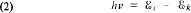Thus arises a line spectrum—the principal distinctive feature of atomic spectra. Bohr obtained the correct formula for the frequencies of the spectral lines of the hydrogen atom (and hydrogen-like atoms); this formula encompassed the aggregate of previously discovered empirical formulas.

The existence of energy levels in atoms was confirmed by the Franck-Hertz experiments (1913–14). It was established that electrons bombarding a gas lose, on collision with atoms, only definite amounts of energy equal to differences between energy levels of the atoms.

Thus, using the quantum constant h, which reflects the duality of light, Bohr demonstrated that this quantity also determines the motion of electrons in the atom (and that the laws of this motion differ significantly from the laws of classical mechanics). This fact was later explained on the basis of the universality of the wave-particle duality contained in de Broglie’s hypothesis.

The success of Bohr’s theory, like the previous advances in quantum theory, was achieved at the expense of disrupting the logical integrity of the theory: on the one hand, Newtonian mechanics was used, and on the other, artificial quantization rules alien to it and contradictory to classical electrodynamics were brought in. Moreover, Bohr’s theory proved unable to explain the motion of electrons in complex atoms (and even in the helium atom), the origin of molecular bonds, and other phenomena. Nor could Bohr’s “semiclassical” theory answer the question of how an electron moves during a transition from one energy level to another. Further intensive work on the problems of atomic theory led to the conviction that it was impossible to construct logically an orderly theory while retaining the classical picture of the electron’s orbital motion. When it was recognized that the motion of electrons in the atom cannot be described in terms (or concepts) of classical mechanics (like motion along a specific trajectory), it was proposed that the problem of the motion of an electron between levels was incompatible with the nature of the laws governing the behavior of electrons in the atom and that a new theory that would incorporate only quantities applying to the initial and final stationary states of the atom was needed. In 1925, W. Heisenberg succeeded in constructing such a formal scheme, in which certain abstract algebraic quantities—matrices—figured instead of electron coordinates and velocities; the relation between the matrices and observable quantities (energy levels and the intensities of quantum transitions) was given by simple self-consistent rules. Heisenberg’s work was developed by M. Born and P. Jordan. Thus arose matrix mechanics. Soon after the appearance of the Schrödinger equation, the mathematical equivalence of wave mechanics (based on the Schrödinger equation) and matrix mechanics was demonstrated. In 1926, Born gave a probabilistic interpretation of de Broglie waves (see below).

Dirac’s works of this period played a major role in the development of quantum mechanics. Final formulation of quantum mechanics as a consistent physical theory with clear foundations and an orderly mathematical apparatus took place after the appearance of Heisenberg’s work (1927) in which the uncertainty relation was formulated. This is a most important relation which sheds light on the physical significance of the equations of quantum mechanics, on the connection with classical mechanics, and on other fundamental questions and qualitative results of quantum mechanics. This work was continued and generalized in the works of Bohr and Heisenberg.

A detailed analysis of atomic spectra led to the concept (first introduced by G. Uhlenbeck and S. Goudsmit and developed by W. Pauli) that in addition to charge and mass yet another intrinsic characteristic (quantum number)—spin—should be ascribed to the electron. The exclusion principle (the Pauli exclusion principle, see below ), discovered by Pauli in 1925 and of fundamental importance in atomic, molecular, nuclear, and solid-state theory, played an important role.

Within a short time, quantum mechanics was applied successfully to a broad range of phenomena. Theories of atomic spectra, molecular structure, the chemical bond, D. I. Mendeleev’s periodic system, conductivity of metals, and ferromagnetism were devised. These and many other phenomena became, at least qualitatively, comprehensible. Subsequent fundamental development of quantum theory was connected primarily with relativistic quantum mechanics. Nonrelativistic quantum mechanics developed primarily along the lines of encompassing diverse specific problems of the physics of atoms, molecules, solids (metals and semiconductors), plasmas, and the like, while at the same time improving the mathematical apparatus and elaborating the quantitative methods of solving various problems.

Probabilities and waves. Insofar as the laws of quantum mechanics cannot be visualized to the same degree as the laws of classical mechanics, it is useful to trace the development of the ideas forming the foundation of quantum mechanics and only then to formulate its basic propositions. The facts on the basis of which the theory is constructed are not, of course, unique, inasmuch as quantum mechanics describes an extremely broad range of phenomena, and each of these is capable of providing material for its substantiation. We shall proceed from the requirements of simplicity and in close conformity with history.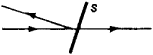Figure 1

Let us consider a very simple experiment in light propagation (Figure 1). A transparent plate S is placed in the path of a beam of light. Some of the light passes through the plate and some is reflected. It is known that light consists of “particles”—photons. What happens to a single photon on impact with the plate? If we set up an experiment (for example, with a beam of light of extremely low intensity) in which the fate of every photon can be traced, then we may satisfy ourselves that on encountering the plate the photon is not split into two photons; its individuality as a particle is retained (otherwise the light would change its frequency, or its color). It turns out that some photons pass through the plate and some are reflected from it. Why? Perhaps there are two different types of photons? Let us set up a control experiment: we place a similar plate in the path of the transmitted light that should contain just one of the two “types” of photons. However, the same picture will be observed: some of the photons will pass through the second plate and some will be reflected. Consequently, under identical conditions identical particles may behave differently. This means that a photon’s behavior on encountering a plate cannot be predicted unambiguously. Determinism does not exist in the sense in which it is construed in classical mechanics during the motion of photons. This conclusion is one of the starting points for resolving the contradiction between the corpuscular and wave properties of particles and constructing a theory of quantum-mechanical phenomena.

The problem of the reflection of light from a transparent plate does not present any difficulty for wave theory: on the basis of the properties of the plate, wave optics unambiguously predicts a correlation between the intensities of the transmitted light and the reflected light. From the corpuscular standpoint, the intensity of the light is proportional to the number of photons. Let N be the total number of photons and N1 and N2, the number of transmitted and the number of reflected photons (N1 + N2 = N). Wave optics defines the ratio N/N2, but naturally nothing can be said of the behavior of a single photon. The reflection of a photon from the plate or its passage through it are random events: some photons pass through the plate and some are reflected from it, but when the number of photons is large the ratio N1/N2 is in agreement with the prediction of wave optics. In quantitative terms, the regularities that are manifested in random events can be described by means of the concept of probability. A photon can pass through a plate with a probability ω and be reflected from it with a probability ω2. When the total number of photons is N, an average of W2N particles will pass through the plate and ω2N2N particles will be reflected. If N is very large, then the average (expected) values of the number of particles coincide precisely with the actual values (although fluctuations exist and classical optics cannot take them into consideration). All optical relations can be translated from the language of intensities into the language of probabilities, and then they will apply to the behavior of a single photon. The probability that one of two alternative (mutually exclusive) events—transmission or reflection—will occur is equal to ω1 + ω2 = 1. This is the addition law of probabilities, which corresponds to the summation of intensities. The probability of passage through two identical plates is equal to ω21 and the probability of passage through the first and reflection from the second is ω2 ω2 (this corresponds to the fact that at the second plate the light that passed through the first plate is divided into transmitted and reflected light in the same proportion as at the first plate). This is the multiplication law of probabilities (which is valid for independent events).

This experiment is not unique to light. Similar experiments with a beam of electrons of other microparticles also demonstrate the unpredictability of the behavior of an individual particle. However, not only direct experiments indicate that in the most general case a probabilistic description of the behavior of microparticles should be used. It is theoretically impossible to imagine that some microparticles can be described probabilistically and others, classically: the interaction of “classical” particles with “quantum” particles would inevitably lead to the introduction of quantum uncertainties and would also make the behavior of “classical” particles unpredictable in the sense of classical determinism.

The prediction of the probabilities of various processes is a possible formulation of the task of quantum mechanics; this contrasts with the task of classical mechanics, which in principle consists in the prediction of completely certain events alone. Of course, a probabilistic description is also permissible in classical mechanics. To obtain a reliable prediction, classical mechanics requires absolutely precise assignment of the initial conditions, that is, of the positions and velocities of all particles forming the system. If the initial conditions are given not precisely but with some degree of uncertainty, the predictions will contain uncertainty, that is, will be to some extent probabilistic in character. Classical statistical physics, which operates with certain averaged quantities, may serve as an example. Therefore, the disparity between the systems of thought of quantum and classical mechanics would not be as great if probabilities were indeed the basic concepts of quantum mechanics. In order to clarify the radical distinction between quantum mechanics and classical mechanics, let us somewhat complicate the light reflection experiment examined above.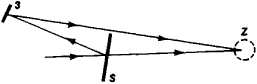Figure 2

Let a reflected beam of light (or microparticles) be rotated by means of a mirror Z and be incident upon the same region A (for example, the same photon detector) as the transmitted beam (Figure 2). It would be natural to expect that in this case the measured intensity is equal to the sum of the intensities of the transmitted and reflected beams. However, it is common knowledge that this is not the case: the intensity may change as a function of the location of the mirror and detector over a broad range and in some cases (when the intensities of the transmitted and reflected light are equal) may vanish (the beams seemingly cancel each other). This is the phenomenon of light interference. What can be said of the behavior of a single photon in an interference experiment? The probability of its incidence on a given detector is changed significantly in contrast to the first experiment and will not be equal to the sum of the probabilities of the photon’s entry into the detector by the first and second paths. Consequently, these two paths are not alternative (otherwise the probabilities would be summed). Hence it follows that the existence of two paths of arrival for the photon from the source to the detector significantly affects the probability distribution, and therefore we cannot say by which path the photon has passed from the source to the detector. We must assume that it could arrive simultaneously by two different paths.

The radical nature of the concepts that arise here must be emphasized. Indeed, it is impossible to conceive of a particle’s simultaneous motion in two paths. Nor does quantum mechanics formulate such a problem. It merely predicts the results of experiments with beams of particles. Let us emphasize that in this case no hypotheses are advanced, but only an interpretation of the wave experiment from the standpoint of corpuscular concepts is given. (Let us recall that we are dealing not only with light but also with any beam of particles, such as electrons.) The result obtained signifies the impossibility of a classical description of the motion of particles in trajectories, and the nonvisualization of a quantum description.

Nonetheless, let us attempt to determine which path the particle followed by setting detectors in its possible paths. Naturally, the particle will be recorded at one site rather than at all possible sites at once. However, as soon as the measurement singles out a specific trajectory of the particle, the interference picture disappears. The probability distribution changes. Both (all) possible trajectories are needed for interference to arise. Thus, the recording of the article’s trajectory changes the conditions in such a way that the two paths are alternative, and as a result the summation of intensities, which would have been obtained in the case of a “classical” particle moving in definite trajectories, is obtained.

An accurate description of the experimental conditions under which a given phenomenon is observed is very important for quantum phenomena. In particular, the conditions also include the measuring instruments. In classical physics it is assumed that, in principle, the role of a measuring instrument can be reduced solely to recording the motion and that the state of the system does not change during measurement. In quantum physics this assumption is invalid: in addition to other factors, the measuring instrument itself participates in the formation of the phenomenon studied in the experiment, and this role must be taken into account. The role of measuring instruments in quantum phenomena was comprehensively analyzed by Bohr and Heisenberg and is closely connected with the uncertainty principle, which will be examined below.

Paying heed to the role of measurements does not mean that in quantum mechanics physical phenomena such as the properties of particles per se are not studied without reference to the instruments. For example, the problems of atomic energy levels, of the scattering of microparticles on collision with each other, and of interference phenomena, all of which are solved by quantum mechanics, are problems of the properties and behavior of particles. The role of the instrument moves to the foreground when specific questions are raised, some of which, as has been found, are meaningless (such as the problem in an interference experiment concerning which trajectory an electron moves along since there is either no trajectory or no interference).

Let us return to the interference experiment. Thus far only a negative assertion has been made: a particle does not move along a specific path, and the probabilities are not summed. A constructive assumption for describing such a situation may be drawn once again from wave optics. In optics, every wave is characterized not only by intensity but also by phase (the intensity is proportional to the square of the amplitude). Both of these two real quantities—the amplitude A and the phase ϕ—are commonly combined into one complex number, which is called the complex amplitude: ψ = Ae. Then, the intensity is equal to I = |ψ|2 = ψ*ψ = A2 = A2, where ψ* is a function that is the complex conjugate of Since the intensity is measured directly, the phase is in no way manifested for a single wave. In the experiment involving the transmission and reflection of light, this is precisely the situation: there are two waves ψ1 and ψ2 but one of them exists only on the right and the other only on the left (see Figure 1); the intensities of these waves are I2 =A22 and I1 = A21, and the phases are not manifested (therefore we were able to deal only with the intensities). In the interference experiment the situation has changed: the wave ψ2 has been directed by means of the mirror to the region where wave ψ1 is located (see Figure 2). The wave field in the region of existence of the two waves is determined in optics by means of the principle of superposition: the waves are superimposed on one another with due consideration of their phases. The sum wave ψ has a complex amplitude equal to the sum of the complex amplitudes of the two waves:The intensity of the sum wave depends on the phase difference ϕ1 - ϕ2 (which is proportional to the difference in the path length of the beams of light along the two trajectories):In particular, when A1 = A2 and cos (ϕ1 – ϕ1) = –1, then |Ψ|2 = 0.

In this example, the simplest case of the addition of amplitudes was considered. In the more general case, the amplitudes may change in magnitude and phase because of a change in conditions (for example, the properties of the mirror), so that the sum wave will have the form

Ψ = C1 Ψ1 + c2Ψ2

where C1 and |c2| are complex numbers:

c1 = |c1|e1c2 = |c2|eiα2

Here, the fundamental essence of the phenomenon does not change, nor does the character of the phenomenon depend on the total intensity. If α is increased by a factor of C, then the intensity will increase by a factor of | C|2, that is, |C|2 will be a common factor in the formula for the distribution of intensities. The number C may be considered complex as well as real, and the physical results do not contain the phase of the number C —it is arbitrary.

Transference of the principle of superposition to quantum mechanics is necessary in order to interpret wave phenomena from the corpuscular point of view. Since quantum mechanics deals with probabilities rather than intensities, a probability amplitude Ψ = Ae must be introduced with the assumption (by analogy with optical waves) that the probability is w = |C|2= Ψ*Ψ. Here C is a number, called the normalization factor, which should be selected such that the total probability for the detection of a particle at all possible points is equal to 1, that is, Σiwi = 1. The factor C is defined only by its modulus; its phase is arbitrary. The normalization factor is important only for determining the absolute probability; relative probabilities are determined by the probability amplitudes in an arbitrary normalization. In quantum mechanics the probability amplitude is also called the wave function.

Probability amplitudes (like optical amplitudes) satisfy the principle of superposition: if and if Ψ1 and Ψ2 are the probability amplitudes of a particle’s passage along the first and second paths, respectively, then the probability amplitude for the case in which both paths are realized should be Ψ = Ψ1 + Ψ2. Hence the phrase “the particle traveled along both paths” acquires wave meaning, and the probability w = |Ψ1 + Ψ2|2 displays interference properties.

The difference in the meaning contained in the principle of superposition in optics (and other wave processes) and in quantum mechanics should be emphasized. The addition (superposition) of ordinary waves is not at variance with visual representations, since each of the waves represents a possible type of oscillation, and the superposition corresponds to the addition of these oscillations at each point. At the same time, quantum-mechanical probability amplitudes describe alternative motions (which are mutually exclusive from the classical standpoint; for example, the waves Ψ1 and Ψ2 correspond to particles entering the detector by two different paths). From the classical point of view, the superposition of these motions is totally incomprehensible. The difficulty of visualizing the quantum-mechanical principle of superposition is manifested herein. The probabilistic interpretation makes it possible to avoid the formal logical contradiction of the quantum-mechanical principle of superposition (the possibility that a particle may travel simultaneously along two paths). Arranging an experiment to determine the path of a particle (see above) leads to a situation in which the particle will travel the first path with a probability | Ψ1 |2 and the second path with a probability | Ψ2 |2. The resulting distribution of the particles on the screen will be determined by the probability | Ψ1 |2 + | Ψ2 |2, that is, the interference will disappear.

Thus, examination of the interference experiment leads to the following conclusion. The probability amplitude, or wave function, of a physical system is the quantity that describes its state in quantum mechanics. The main feature of this quantum-mechanical description is the assumption that the principle of the superposition of states is valid.

The principle of superposition is a fundamental principle of quantum mechanics. In its general form it asserts that if under given conditions different quantum states of a particle (or system of particles) to which the wave functions Ψ1, Ψ2, …, Ψi, … correspond are possible, then there also exists a state described by the wave function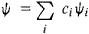where ci, are arbitrary complex numbers. If the Ψi describe the different possible states, then | ci |2 defines the probability that the system is in a state that has the wave function Ψi and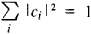De Broglie waves and the uncertainty relation. One of the principal tasks of quantum mechanics is to find the wave function that corresponds to a given state of the system being studied. Let us examine the solution to this problem by using the very simple but important case of a free-moving particle. According to de Broglie, a wave having the wavelength λ = h/p is associated with a free particle having momentum p. This means that the wave function of a free particle, Ψ (x) —the de Broglie wave—should be a function of the coordinate x such that when λ changes by Ψ, the wave function v|/ reverts to its previous value. The function eix has this property. If we introduce the quantity k = 2π/λ, called the wave number, then the de Broglie relation assumes the form p = (h/2π)k = ℏΔk. Thus, if a particle has a definite momentum p, its state is described by the wave functionwhere C is a constant complex number. This wave function has a remarkable property: the square of its modulus | Ψ |2 does not depend on x, that is, the probability of finding the particle described by such a wave function is the same for any point in space. In other words, a particle with a precisely defined momentum is totally nonlocalized. Of course, this is an idealization— totally nonlocalized particles do not exist. But a wave with a precisely defined wavelength, and consequently with a precisely defined momentum of the particle, is an idealization to the same extent. Therefore, it is more accurate to state this in a different manner: the better defined a particle’s momentum, the less defined its position (coordinates). Herein lies the uncertainty principle that is specific to quantum mechanics. In order to obtain a quantitative expression of this principle—the uncertainty relation— let us examine a state that represents the superposition of some or, more accurately, of an infinitely large number of de Broglie waves having nearly the same wave number, that is, lying in a small interval Δk. The wave function Ψ(x) (called a wave packet), which is obtained as a result of superposition, has the following character: near some fixed value x0 all amplitudes are summed, but at a distance from x0 (| xx0 | ≫ λ) they will cancel each other out because of the large diversity in phases. It turns out that such a wave function is actually concentrated in a region Δx wide, which is inversely proportional to the interval Δk, that is, Δx ≈ 1/Δk, or ΔxΔp ≈ ℏ (where Δp = ℏΔk is the uncertainty in the particle’s momentum). This relation is the Heisenberg uncertainty relation.

Any function Ψ (x) can be represented mathematically as the superposition of simple periodic waves—this is the well-known Fourier transformation on the basis of whose properties the uncertainty relation between Δx and Δk can be found in a rigorous mathematical manner. The precise relation has the form of the inequality ΔxΔk ≥ 1/2, or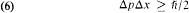where the uncertainties Δp and Δx are understood to be the dispersions, that is, the mean square deviations of the momentum and coordinate from their average values. The physical interpretation of relation (6) is that (in contrast to classical mechanics) there exists no state in which the coordinate and momentum of the particle simultaneously have precise values. The extent of the uncertainties of these quantities is given by Planck’s constant ℏ, and herein lies the important meaning of this universal constant. If uncertainties connected by the Heisen-berg relation can be considered small in a given problem and can be neglected, the motion of the particle will be described by the laws of classical mechanics (as motion along a definite trajectory).

The uncertainty principle is a fundamental principle of quantum mechanics, and it defines the physical content and structure of the mathematical apparatus of quantum mechanics. Moreover, it plays a major heuristic role, since many results of quantum mechanics can be derived and understood on the basis of a combination of the laws of classical mechanics and the uncertainty relation. The problem of the stability of the atom, which was discussed above, is an important example. Let us examine this problem for the hydrogen atom. Let the electron move with a velocity v in a circular orbit of radius r around the nucleus (a proton). According to Coulomb’s law, the forces attracting the electron to the nucleus is equal to e2/r2, where e is the absolute value of the electron’s charge, and the centripetal acceleration is equal to v2/r. According to Newton’s second law, mv2/r = e2/r2, where m is the mass of the electron. From here it follows that the radius of the orbit r = e2/mv2 may be made arbitrarily small if the velocity v is made sufficiently large. But in quantum mechanics the uncertainty relation must be fulfilled. If we assume the uncertainty of the electron’s position is within the limits of the radius of its orbit r and the uncertainty of its velocity within the limits of v, that is, the momentum within the limits Δp = mv, then the uncertainty relation will assume the form mvr ≥ ℏ. By using the relationship between v and r, as defined by Newton’s law, we obtain ve2/ℏ and r ≥ ℏ2/me2. Consequently, it is impossible for the electron to move in an orbit with a radius less than r0 = ℏ2/me2 ≈ 0.5 × 10-8 cm, and the electron cannot fall into the nucleus—the atom is stable. The quantity r0 is the radius of the hydrogen atom (the “Bohr radius”). The greatest possible binding energy of the atom ε0 (equal to the total energy of the electron in the atom, that is, the sum of the kinetic energy mv2/2 and the potential energy —e2/r0, which gives ε0, = —e2/2r0 ≈ —13.6 electron volts), which determines its minimum energy— the energy of the ground state—corresponds to this radius.

Thus, quantum-mechanical concepts first provided the possibility of theoretically estimating the dimensions of the atom (by expressing its radius in terms of the universal constant ℏ, m, and e). The “smallness” of atomic dimensions proves to be related to the “smallness” of the constant ℏ.

It is noteworthy that modern concepts of atoms that have well-defined stable states prove to be closer to the concepts of ancient atomists than the planetary model of the atom, which is based on the laws of classical mechanics and allows the electron to be located at any distance from the nucleus.

A rigorous solution to the problem of the electron’s motion in the hydrogen atom is obtained from the quantum-mechanical equation of motion—the Schrödinger equation (see below); solution of the Schrödinger equation gives the wave function which Ψ, describes the state of an electron located in the region of attraction of the nucleus. But even without knowing the explicit form of Ψ we may assert that this wave function represents a superposition of de Broglie waves that corresponds to the electron’s localization in a region having the dimension ≥r0 and to the momentum range Δp ~ ℏ/r0.

The uncertainty relation also makes it possible to understand the stability of molecules and to estimate their dimensions and minimal energy. In addition, it explains the existence of a substance (helium) which, at normal pressure, is not converted into the solid state at any temperature and provides qualitative models of the structure and size of the nucleus.

The existence of energy levels is a characteristic quantum phenomenon inherent in all physical systems and does not stem directly from the uncertainty relation. It will be shown below that the discreteness of the energy levels of a bound system can be explained on the basis of the Schrodinger equation; let us observe only that the possible discrete energy values (energy levels) εn > ε0 correspond to excited states of a quantum-mechanical system.

Stationary Schrodinger equation. De Broglie waves describe the state of a particle only in the case of free motion. If a field of forces with a potential energy v (also called the potential) that depends on the coordinates of a particle acts on the particle, then the wave function of the particle Ψ is given by a differential equation obtained by the following generalization of the de Broglie hypothesis. For the case in which the motion of a particle with a given energy ε takes place in one dimension (along the x-axis), an equation satisfied by the de Broglie wave (5) may be written in the form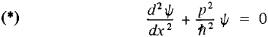where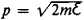is the momentum of a free-moving particle of mass m. If a particle with an energy ε moves in a potential field V(x) that is independent of time, the square of its momentum (which is defined by the law of conservation of energy) is equal to p2 = 2m[ε — V(x)]. Therefore, the equation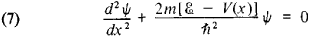is the simplest generalization of equation (*). This is called the stationary (time-independent) Schrodinger equation and is one of the basic equations of quantum mechanics. The solution of this equation depends on the type of forces, that is, on the form of the potential V(x). Let us examine several typical cases.Figure 3

(1) V = constant, ε > V. The solution is the de Broglie wavev Ψ = Ceikx, where ℏ2k2/2m = p2/2m = ε - V is the kineticenergy of the particle.

(2)A potential wall:

V = 0 when x < 0

y = Vl < 0 when x > 0

If the total energy of the particle is greater than the height of the wall, that is, ε > V1, and if the particle moves from left to right (Figure 3), then the solution to equation (7) in the region x < 0 has the form of two de Broglie waves—the incident wave and the reflected wave: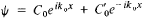where(a wave with a wave number k = — k0 corresponds to motion from right to left with the same momentum p0); when x > 0, the solution has the form of a traveling de Broglie wave:

Ψ = C1eik1x

where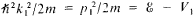The ratios |C1/C0 |2 and | C0/C0|2 give the probabilities of the particle passing over the wall and being reflected from it. The existence of the reflection is a uniquely quantum-mechanical (wave) phenomenon (analogous to the partial reflection of a light wave from the interface between two transparent media): a “classical” particle passes above the bar rier, and onlyits momentum is reduced to the value of.

If the energy of the particle is less than the height of the wall, ε < V (Figure 4, a), then the kinetic energy of the particle ε — V in the domain x > 0 is negative. In classical mechanics this is impossible, and the particle does not reach this region of space —it is reflected from the potential wall. Wave motion is of a different character. A negative value of k2 (p2/2m = ℏ2k2/2 m< 0) means that k is a purely imaginary quantity, k= iK, where K is real. Therefore, the wave eikx is converted into e-Kk, that is, the oscillatory mode is replaced by a damped mode (K > 0); otherwise as x increases a physically meaningless, unlimited increase of the wave would result. This phenomenon is well known in the theory of oscillations. The qualitative behavior of the wave function Ψ (x), or more accurately of its real part, is represented beneath the energy diagram in Figure 4, a (and Figure 4, b).Figure 4

(3) Two regions containing no forces are separated by a rectangular potential barrier V, and the particle moves toward the barrier from the left with an energy ε < V (Figure 4, b). According to classical mechanics, the particle will be reflected from the barrier; according to quantum mechanics, the wave function is not equal to zero even within the barrier and to the right once again will have the form of a de Broglie wave with the same momentum (that is, with the same frequency but, of course, with a smaller amplitude). Consequently, the particle can pass through the barrier. The smaller the width and height of the barrier (the smaller the difference V — ε), the greater the coefficient (or probability) of transmission. This typically quantum-mechanical effect, called the tunnel effect, is of great importance in the practical applications of quantum mechanics. It explains, for example, the phenomenon of alpha decay—the emission of a-particles (helium nuclei) from radioactive nuclei. In thermonuclear reactions, which occur at temperatures of tens and hundreds of millions of degrees, most of the reacting nuclei overcome electrostatic (Coulomb) repulsion and approach each other to distances of the order of the range of nuclear forces as a result of tunneling (subbarrier) transitions. The possibility of tunneling transitions also explains spontaneous electron emission—the phenomenon of the emission of electrons from a metal in an electric field—contact phenomena in metals and semiconductors, and many other phenomena.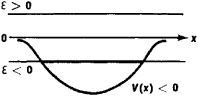Figure 5

Energy levels. Let us examine the behavior of a particle in the field of an arbitrary potential well (Figure 5). Let the potential be nonzero in some finite region with V < 0 (attractive force). Here, both the classical and quantum motions are highly dependent on whether the total energy ε of the particle is positive or negative. When ε > 0, a “classical” particle passes over and moves away from the well. The quantum-mechanical motion differs from the classical motion in that partial reflection of the wave from the well occurs in the former; also, the possible energy levels are in no way limited—the particle’s energy has a continuous spectrum. When ε < 0, the particle proves to be “locked” within the well. In classical mechanics this limitation in the extent of the motion is absolute and possible for any values ε < 0. In quantum mechanics the situation changes significantly. The wave function must be attenuated in both directions from the well, that is, it assumes the form e-K|x|. However, a solution that satisfies this condition does not exist at all values of ε but only at certain discrete values. The number of such discrete values of εn may be finite or infinite but is always denumerable, that is, can be counted, and always has a least value ε0 (which lies above the bottom of the potential well); the number n of a solution is called the quantum number. In this case it is said that the energy of the system has a discrete spectrum. The discreteness of the permissible energy values of a system (or of the corresponding frequencies ω = εn/ℏ where ω = 2πv is the angular frequency) is a typical wave phenomenon. Analogues to it can be observed in classical physics, where wave motion takes place in a bounded region. Thus, the oscillation frequencies of a string or the frequencies of electromagnetic waves in a cavity resonator are discrete and are determined by the dimensions and properties of the boundaries of the region in which the oscillations take place. Indeed, the Schrodinger equation is mathematically similar to the corresponding equations for a vibrating string or resonator.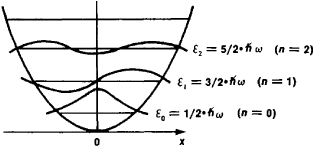Figure 6

Let us illustrate a discrete energy spectrum by using a quantum oscillator as an example. The distance of the particle from the state of equilibrium is plotted along the x-axis in Figure 6. The curve (a parabola) represents the potential energy of the particle. In this case, the particle is “locked” within the well at all energies, and therefore the energy spectrum is discrete. The horizontal straight lines represent the particle’s energy levels. The energy of the lowest level is ε0 = ℏω/2; this is the lowest energy level compatible with the uncertainty relation: if the particle were located at the bottom of the well (ε = 0), this would signify precise equilibrium, for which both x = 0 and p = 0, which is impossible according to the uncertainty principle. Subsequent, higher energy levels of the oscillator lie at equal distances at intervals of ℏω; the formula for the energy of the nth level is: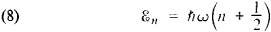The conventional representation of the wave function of a given state is presented in Figure 6 about each horizontal line. It is characteristic that the number of nodes of the wave function (that is, the number of passages through 0) is equal to the quantum number n of the energy level. On the other side of the well (beyond the point of intersection of the level and the potential curve), the wave function attenuates rapidly in accordance with the foregoing reasons.

In the general case, every quantum-mechanical system is characterized by its own energy spectrum. Depending on the type of potential (or more accurately, on the nature of the interaction in the system), the energy spectrum may be discrete (as in an oscillator), continuous (as in a free particle, its kinetic energy may have any positive value), or partially discrete and partially continuous (for example, the levels of an atom are discrete at excitation energies less than the ionization energy but are continuous at higher energies).

Especially important is the case occurring in atoms, molecules, nuclei, and other systems when the lowest energy level corresponding to the ground state of the system lies in the region of the discrete spectrum, and consequently the ground state is separated from the first excited state by an energy gap. As a result, the internal structure of the system is not manifested until an energy exchange during interactions with other systems exceeds a certain value—the width of the energy gap. Therefore, when there is limited energy exchange, a complex system (such as a nucleus or atom) behaves like a structureless particle (a material particle). This is of fundamental importance for understanding, for example, thermal motion. Thus, at energies of thermal motion lower than the excitation energy of the atomic levels, the electrons of atoms cannot participate in the exchange of energy and do not contribute to heat capacity.

Time-dependent Schrödinger equation. Thus far we have examined only the possible quantum states of a system and have not discussed the evolution of the system with time (its dynamics), which is determined by the dependence of the wave function on time. A full solution of the problems of quantum mechanics should give the wave function Ψ as a function of the coordinates and of the time t. For one-dimensional motion it is defined by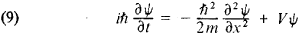which is the equation of motion in quantum mechanics. This equation is called the time-dependent Schrodinger equation. It also is valid for the case when the potential energy is time-dependent: V = V(x, t).

The functions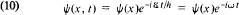are partial solutions to equation (9). Here ε is the particle’s energy and Ψ(x) satisfies the stationary Schrödinger equation (7); for free motion, Ψ(x) is a de Broglie wave eikx.

The wave functions (10) have an important property, namely, that the corresponding probability distributions are time-independent, since |Ψ(x, t)|2 = |Ψ(x)|2. Therefore, the states described by such wave functions are called stationary; they play a special role in applications of quantum mechanics.

The general solution to the time-dependent Schrodinger equation is a superposition of stationary states. In this general (non-stationary) case, when the probabilities change significantly with time, the energy ε does not have a specific value. Thus, if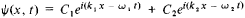then ε = ℏω1 with a probability |C1|2 and ε = ℏω2 with a probability |C2|2. For energy and time there exists the uncertainty relation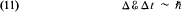where Δε is the energy dispersion and Δt, the time interval over which the energy can be measured.

Three-dimensional motion. Thus far we have examined, for the sake of simplicity, one-dimensional motion. Generalization to the motion of a particle in three dimensions does not contain fundamentally new elements. In this case, the wave function depends on the three coordinates x, y, and z (and time): Ψ = Ψ(x, y, z, t) and the de Broglie wave has the form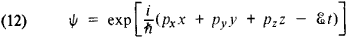where pxpy, and pz are the three projections of the momentum on the coordinate axes and ε = (px2 + py2 + pz2)/2m. Correspondingly, there are three uncertainty relations:The time-dependent Schrodinger equation has the form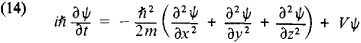This equation commonly is written in the symbolic form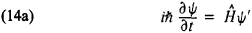where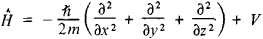is a differential operator called the Hamiltonian operator, or the Hamiltonian.

The stationary solution to equation (14) is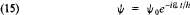where Ψ0 is the solution to the Schrodinger equation for stationary states: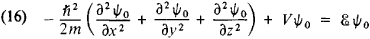or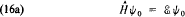In the case of three-dimensional motion, the energy spectrum may also be continuous and discrete. The case in which several different states have identical energy is also possible; such states are said to be degenerate. In the case of a continuous spectrum, a particle moves to an infinitely great distance from the center of forces. But in contrast to one-dimensional motion (for which there are only two possibilities, transmission or reflection), during three-dimensional motion a particle can move away from the center at an arbitrary angle to the direction of the original motion, that is, it may be scattered. The wave function of the particle now is a superposition not of two but of an infinite number of de Broglie waves propagated in all possible directions. It is convenient to describe the scattered particles in spherical coordinates, that is, to define their position by their distance r from the center (by the radius) and by two angles—the latitude Θ and the azimuth Ф. At large distances r from the center of forces, the corresponding wave function has the form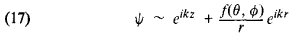The first term, which is proportional to a de Broglie wave propagating along the z-axis describes the incident particles, while the second, which is proportional to a “radial de Broglie wave,” describes the scattered particles. The function f(Θ, Ф) is called the scattering amplitude; it defines the “differential scattering cross section” , which characterizes the probability of scattering at given angles: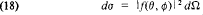where dΩ is the solid angle element into which the scattering takes place.

A discrete energy spectrum arises, as in the case of one-dimensional motion, when a particle is located within a potential well. The energy levels are specified, in contrast to one-dimensional motion, by three, not one, quantum numbers. The problem of motion in a field of central attractive forces is of the greatest importance. In this case, it is also convenient to use spherical coordinates.

Angular momentum. The angular part of motion (rotation) is given in quantum mechanics as in classical mechanics by assigning an angular momentum, which is conserved during motion in a field of central forces. In contrast to classical mechanics, the angular momentum in quantum mechanics has a discrete spectrum, that is, can assume only certain values. This can be demonstrated by using as an example azimuthal motion—rotation around a fixed axis (we shall use the z-axis). In this case, the wave function has the form of an “angular de Broglie wave” eimФ, where Ф is the azimuth and the number m is related to the angular momentum Mz in the same manner as the wave number k is related to the linear momentum p in a plane de Broglie wave, that is, m = Mz/ℏ. Since the angles Ф and Ф + 2π describe the same position, when Ф changes by 2π the wave function should return to its former value. Thus it follows that m can assume only integral values: m = 0, ±1, ±2, …, that is, the angular momentum must equal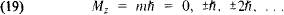Rotation about the z-axis is only a part of the angular motion (it is the projection of the motion on the xy plane), and Mz is not the total angular momentum but only its component along the z-axis. In order to know the total angular momentum, it is necessary to determine its other two components. However, in quantum mechanics all three components of the angular momentum cannot be given simultaneously with precision. Indeed, a component of angular momentum contains the product of a component of linear momentum and the corresponding radius (the coordinate perpendicular to the linear momentum), and all the components of linear momentum and all the radii cannot, according to the uncertainty relations (13), simultaneously have precise values. It turns out that in addition to the projection Mz of the angular momentum on the z-axis (defined by m), the total angular momentum Af, defined by the integer /, can be accurately given simultaneously: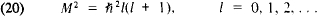Thus, angular motion gives two quantum numbers—l and m. The number l is called the orbital quantum number, and the value of the particle’s energy can depend on it (as it depends in classical mechanics on the size of the orbit). The number m is called the magnetic quantum number and, for a given l, can assume the values m = 0, ¿1, ¿2, …, ±l, for a total of 2l + 1 values. The energy does not depend on m, since the value of m itself depends on the selection of the z-axis, and the field has spherical symmetry. Therefore, a level with the quantum number l has a (2l + 1)-fold degeneracy. The energy of the level begins to depend on m only when the spherical symmetry is broken, for example when the system is placed in a magnetic field (the Zeeman effect).

For a given momentum, the radial motion is similar to one-dimensional motion with the difference that the rotation induces centrifugal forces. They are taken into account by introducing (in addition to the ordinary potential) a centrifugal potential, which has the form M2/2m r2 as in classical mechanics (here m is the mass of the particle). The square of the angular momentum M2 must be replaced by the quantity ℏ2l(l + 1). The solution to the Schrödinger equation for the radial part of an atom’s wave function gives its energy levels and introduces a third quantum number—the radial quantum number nr or the principal quantum number n, which are related by the equation n = nr + l + 1, where nr = 0, 1, 2, … and n = 1, 2, 3, …. In particular, for the motion of an electron in the Coulomb field of a nucleus with charge Ze (a hydrogen-like atom), the energy levels are defined by the formula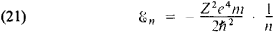that is, the energy depends only on the principal quantum number n. For manyelectron atoms, in which every electron moves not only in the field of the nucleus but also in the field of the other electrons, the energy levels also depend on l.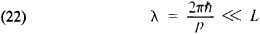In this case the quantum-mechanical laws of motion approximate the classical laws of motion for certain trajectories, just as under similar conditions the laws of wave optics become the laws of geometric optics (which describes the propagation of light by means of rays). Equation (22), the condition for a short de Broglie wavelength, signifies that pL ≫ ℏ, where pL is equal in order of magnitude to the classical action for the system. Under these conditions, the quantum of action ℏ may be considered to be a very small quantity, that is, the conversion of quantum-mechnical laws into classical laws is formally accomplished as ℏ —→ 0. At this limit, all specific quantum-mechanical phenomena disappear, for example the probability of the tunnel effect is zero.

Spin. In quantum mechanics a particle (both a complex particle such as a nucleus and an elementary particle such as an electron) can have an intrinsic angular momentum, which is called the particle’s spin. This means that a quantum number (s) analogous to the orbital quantum number l can be assigned to the particle. The square of the intrinsic angular momentum has the magnitude 2s(s + l), and the component of the angular momentum in a given direction may assume 2s + 1 values from -ℏs to +ℏs at an interval of ℏ. Thus, the state of the particle is (2s + 1)-fold degenerate. Therefore, the de Broglie wave of a particle having spin is analogous to a polarized wave: for a given frequency and wavelength it has 2s + 1 polarizations. The number of such polarizations can be any integer, that is, the spin quantum number s may be either an integer (0, 1, 2, …) or a half-integer (1/2, 3/2, 5/2, …). The spin of the electron, the proton, and the neutron is equal to 1/2 (in units of ℏ). The spin of nuclei consisting of an even number of nucleons (protons and neutrons) is an integer or zero, while the spin of nuclei consisting of an odd number of nucleons is a half-integer. It should be noted that for the photon the relation between the number of polarizations and the spin (which is equal to 1) is different: the photon has no rest mass, and (as relativistic quantum mechanics shows) for such particles the number of polarizations is equal to 2 (and not to 2s + 1 = 3).

Many-particle systems; identical particles. The quantum-mechanical equation of motion for a system of N particles is obtained by appropriate generalization of the Schrödinger equation for a single particle. It contains a potential energy that depends on the coordinates of all N particles and includes both the effect of an external field on them and the interaction between the particles themselves. The wave function is also a function of the coordinates of all particles. It may be considered as a wave in 3A-dimensional space; consequently, the visual analogy with wave propagation in ordinary space is lost. But this now is unimportant inasmuch as the meaning of the wave function as a probability amplitude is known.

If quantum-mechanical systems consist of identical particles, then a unique phenomenon that has no analogy in classical mechanics is observed for them. In classical mechanics the case of identical particles also has a certain peculiarity. For example, let two identical “classical” particles collide (the first moving from the left, the second from the right) and let them fly apart after the collision in different directions (for example, the first can fly upward, the second downward). It is of no consequence to the result of the collision which particle went upward, for example, since the particles are identical; in practice it is necessary to consider both possibilities (Figure 7, a and 7, b). However, in classical mechanics these two processes can be distinguished in principle, since the trajectories of the particles can be traced during the collision. In quantum mechanics there are no trajectories in the strict sense of the word, and both particles pass through the region of collision with some uncertainty, with “diffuse trajectories” (Figure 7, c). In the process of collision the diffuse regions overlap and it is impossible, even in principle, to distinguish these two cases of scattering. Consequently, identical particles become totally indistinguishable. It is meaningless to speak of two different cases of scattering, as there is just one—one particle went upward, the other downward; the particles have no individualitv.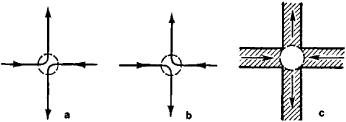Figure 7

This quantum-mechanical principle of the indistinguishability of identical particles may be formulated mathematically in the language of wave functions. The probability of detection of two particles at given points in space is determined by the square of the modulus of the wave function, which depends on the coordinates of both particles, |Ψ(1, 2) |2, where 1 and 2 stand for the set of coordinates (including spin) of the first and second particles, respectively. The identity of the particles requires that when particles 1 and 2 are interchanged, the probabilities remain the same, that is,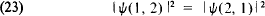Hence, it follows that there may be two cases: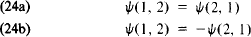If, when the particles are interchanged the wave function does not change sign, then it is called a symmetrical function [case (24a)], and if it changes sign, it is antisymmetrical [case (24b)]. Since all interactions of identical particles are symmetrical with respect to the variables 1 and 2, the properties of the symmetry or antisymmetry of a wave function are conserved in time.

In a system consisting of an arbitrary number of identical particles, symmetry or antisymmetry with respect to the interchange of any pair of particles should exist. Therefore, the property of symmetry or antisymmetry is a characteristic feature of this type of particle. Correspondingly, all particles are divided into two classes: particles with symmetrical wave functions, called bosons, and particles with antisymmetric functions, called fermions. There is a relation between the value of the spin of particles and the symmetry of their wave functions: particles with integral spin are bosons, and particles with half-integral spin are fermions (for the relation between spin and statistics, see below). This rule originally was established empirically and later was proved theoretically by Pauli (it is one of the basic theorems of relativistic quantum mechanics). In particular, electrons, protons, and neutrons are fermions, while photons, pi-mesons, and K-mesons are bosons. Complex particles consisting of fermions are fermions if they consist of an odd number of particles and are bosons if they consist of an even number of particles; atomic nuclei, for example, have these properties.

The symmetry properties of the wave function essentially determine the statistical properties of a system. For example, let noninteracting identical particles exist under identical external conditions (for example, in an external field). The state of such a system can be given by assigning occupation numbers—the numbers of particles in each given (individual) state, that is, particles with the same set of quantum numbers. But if identical particles have the same quantum numbers, their wave function is symmetrical with respect to the interchange of particles. Hence, it follows that two identical fermions belonging to one system cannot be in the same state, since for fermions the wave function must be antisymmetrical. This property is called the Pauli exclusion principle. Thus, the occupation numbers for fermions can assume only the values 0 or 1. Since electrons are fermions, the Pauli principle significantly affects the behavior of electrons in atoms, metals, and elsewhere. For bosons (which have a symmetrical wave function) the occupation numbers can assume arbitrary integral values. Therefore, with consideration for the quantum-mechanical properties of identical particles, there exist two types of particle statistics: Fermi-Dirac statistics for fermions and Bose-Einstein statistics for bosons. An electron gas in a metal is an example of a system consisting of fermions (a Fermi system), while a photon gas (that is, equilibriated electromagnetic radiation) and liquid 4He are examples of Bose systems.

The Pauli principle is definitive for an understanding of the structure of Mendeleev’s periodic table of the elements. In a complex atom there may exist at each energy level a number of electrons equal to the degree of degeneracy of this level (equal to the number of different states with an identical energy). The degree of degeneracy depends on the orbital quantum number and on the spin of the electron; it is equal to

(2l + 1) (2s + 1) = 2(2l + 1)

Thus arises the concept of the electron shells of the atom, which correspond to the periods in Mendeleev’s table of the elements (seeATOM).

Exchange interaction; the molecule. A molecule is a system of nuclei and electrons between which electrical (Coulomb) forces (of attraction and repulsion) act. Since nuclei are much heavier than electrons, the electrons move much more rapidly and form a certain distribution of negative charge, in the field of which are located the nuclei. In classical mechanics and electrostatics it is proved that a system of this type does not have stable equilibrium. Therefore, even if the stability of atoms is assumed (an assumption that cannot, as indicated above, be explained on the basis of the laws of classical physics), it is impossible to explain the stability of molecules without specifically quantum-mechanical principles. The existence of molecules consisting of identical atoms, that is, with a covalent chemical bond (such as the simplest molecule—H2), is especially incomprehensible from the standpoint of classical concepts. It has been shown that the property of the antisymmetry of the electron wave function so changes the character of the interaction of electrons located on different nuclei that the occurrence of such a bond becomes possible.

Let us examine as an example the hydrogen molecule H2, which consists of two protons and two electrons. The wave function of this system is a product of two functions, one of which depends only on the coordinates and the other, only on the spin variables of the two electrons. If the total spin of the two electrons is equal to zero (the spins antiparallel), the spin function is antisymmetrical with respect to the interchange of the spin variables of the electrons. Consequently, in order for the total wave function to be antisymmetrical in accordance with the Pauli principle, the coordinate function must be symmetrical with respect to the interchange of the coordinates of both electrons. This means that the coordinate part of the wave function has the form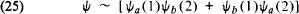where Ψa(i) and Ψb(i) are the wave functions of the rth electron (i= 1, 2) at the nuclei a and b, respectively.

The Coulomb interaction is proportional to the density of the electric charge p = e |Ψ|2 = eΨ*Ψ. If the symmetrical properties of the coordinate wave function (25) are taken into account, in addition to the density of the ordinary type

e |Ψa(1)|2|Ψb(2)|2e |a(1)|2|Ψb(2)|2

which correspond to the motion of single electrons about different nuclei, a density of the following type appears:

e*Ψa(1)Ψb(1)Ψb*(2)Ψa(2)

e*Ψa(1)Ψa(1)Ψa*(2)Ψb(2)

This is called the exchange density, since it appears to arise from an exchange of the electrons between the two atoms. It is this exchange density, which leads to an increase in the density of the negative charge between the two positively charged nuclei, that ensures the stability of the molecule in the case of a covalent chemical bond.

It is obvious that when the total spin of the two electrons is equal to 1, the coordinate part of the wave function is antisymmetrical, that is, a minus sign precedes the second term in (25), and the exchange density has a negative sign. This means that the exchange density will lower the density of the negative electric charge between the nuclei, that is, will lead to additional repulsion of the nuclei.

Thus, the symmetry of the wave function leads to “additional” exchange interaction. The dependence of the exchange interaction on the spins of the electrons is characteristic. The spins do not participate directly in the interaction; electrical forces that depend only on the distance between charges are the source of the interaction. But, depending on the orientation of the spins, a wave function that is antisymmetrical with respect to the complete interchange of the two electrons (together with their spins) may be symmetrical or antisymmetrical with respect to the exchange of the positions of the electrons (their coordinates) alone. The sign of the exchange density, and correspondingly the effective attraction or repulsion of the particles as a result of the exchange interaction, depends on the type of symmetry of the coordinate part of the wave function. Thus, although not participating directly in the dynamics of the interaction, the spins of the electrons actually determine the chemical bond by virtue of the specific quantum-mechanical properties of the identical particles.

Exchange interaction plays a significant role in many phenomena. For example, it explains ferromagnetism: as a result of exchange interaction the spin moments and consequently the magnetic moments of the atoms of a ferromagnetic are aligned parallel to each other. Numerous phenomena in condensed phases (such as liquids or solids) are closely related to the statistics of the particles forming them and to exchange interaction. The wave function antisymmetry for fermions leads to the fact that when the density is high, fermions effectively repel each other (even if no forces act between them). At the same time, attractive forces arise, as it were, between bosons that are described by symmetrical wave functions: the more bosons in some state, the greater the probability that other bosons in the system will pass into this state. (For example, such effects underlie the phenomena of superfluidity and superconductivity and the operating principle of quantum generators and quantum amplifiers.)

Mathematical scheme of quantum mechanics. Nonrelativistic quantum mechanics can be constructed on the basis of a few formal principles. The mathematical apparatus of quantum mechanics is logically faultless and elegant. Its precise rules establish the relation between the elements of the mathematical scheme and physical quantities.

The quantum state is the first basic concept of quantum mechanics. The selection of the mathematical apparatus of quantum mechanics is dictated by the physical principle of superposition of quantum states, which stems from the wave properties of particles. According to this principle, the superposition of any possible state of a system with arbitrary (complex) coefficients is also a possible state of the system.

The objects for which the concepts of addition and multiplication by a complex number are defined are called vectors. Thus, the principle of superposition requires that the state of a system be described by some vector—the state vector (with which the concept of the probability amplitude, or wave function, is closely connected), which is an element of the linear “state space.” This makes it possible to use the mathematical apparatus developed for linear (vector) spaces. The state vector is designated as |Ψ› after P. Dirac.

Apart from addition and multiplication by a complex number, the vector |Ψ› may undergo two other operations. First, it can be projected onto another vector, that is, the scalar product of |Ψ› and any other state vector |Ψ’› may be formed; this is designated as ‹Ψ’ |Ψ› and is a complex number, such that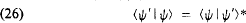The scalar product of the vector |Ψ› and itself, ‹ΨΨ›, is a positive number; it defines the length (magnitude) of the vector. It is convenient to select the length of a state vector equal to 1; its common phase factor is arbitrary. Different states differ from each other in the direction of the state vector in the state space.

Second, we may examine the operation of the transition from the vector |Ψ› to another vector |Ψ’› (or may carry out the transformation |Ψ›. This operation may be written symbolically as the result of the operation on the vector |Ψ› of some linear operator L:Here, the vector |Ψ’› may differ from |Ψ› in “length” and “direction.” Linear operators are of special importance in quantum mechanics by virtue of the principle of superposition of states; as a result of the action of a linear operator on the superposition of the arbitrary vectors |Ψ› and |Ψ›2, superposition of the transformed vectors is obtained: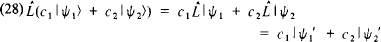The vectors |Ψ› = |Ψλ›, for which |Ψ’› coincides in direction with |Ψ›, that is,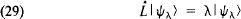play an important role for the operator L. The vectors |Ψ› are called the eigenvectors of operator L, and the numbers λ are called its eigenvalues. The eigenvectors |Ψλ› are commonly designated simply as |λ›, that is, |Ψ› = |λ›. The eigenvalues λ form a discrete series of numbers (then it is said that the operator L has a discrete spectrum), a continuous set (a continuous spectrum), or a partially discrete, partially continuous spectrum.

Linear Hermitian operators constitute a class of operators that is very important for quantum mechanics. The eigenvalues λ of the Hermitian operator L are real. The eigenvectors of a Hermitian operator that belong to different eigenvalues are orthogonal to one another, that is,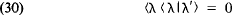Using these vectors, it is possible to construct an orthogonal basis (“Cartesian coordinate axes”) in the state space. It is convenient to normalize these basis vectors to 1, ‹λ|λ› = 1. An arbitrary vector |Ψ› may be resolved with respect to this basis: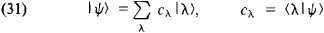Here,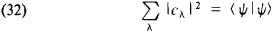which is equivalent to the Pythagorean theorem; if πψ› is normalized for 1, then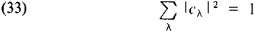The existence for every physical quantity of some isolated states of the system in which this quantity assumes a certain definite (unique) value is of fundamental importance for the construction of the mathematical apparatus of quantum mechanics. This property is essentially the definition of a measurable (physical) quantity, and the states in which the physical quantity has a defined value are called the eigenstates of this quantity.

According to the principle of superposition, any state of a system can be represented in the form of a superposition of the eigenstates of some physical quantity. The possibility of such representation is mathematically analogous to the possibility of expansion of an arbitrary vector in the eigenvectors of a linear Hermitian operator. In accordance with this, in quantum mechanics a linear Hermitian operator L is placed in correspondence to every physical quantity or observable L (such as a coordinate, momentum, angular momentum, or energy). The eigenvalue λ of the operator L is interpreted as the possible values of the physical quantity L that are manifested during measurements. If the state vector |Ψ› is an eigenvector of the operator L, then the physical quantity L has a definite value. Otherwise, L assumes different values of λ with a probability | cλ |2, where cλ is the coefficient of expansion of |Ψ› with respect to |λ› :The coefficient cλ = ‹λ|Ψ› in the expansion of |Ψ› in the basis |λ› is also called the wave function in the λ -representa-tion. In particular, the wave function Ψ(x) is the coefficient of expansion of |Ψ› with respect to the eigenvectors of the coordinate operator x, Ψ(x) = ‹ x |Ψ›.

The average value L of the observed L in a given state is determined by the coefficient according to the general relation between the probability and the average value: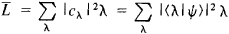The value of L can be found directly through the operator L and the state vector |Ψ› (without determining the coefficients ) from the formula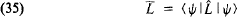The form of the linear Hermitian operators corresponding to such physical quantities as momentum, angular momentum, and energy is determined, on the basis of the general principles for defining these quantities and the correspondence principle, which requires that in the limit ℏ → 0 the physical quantities under consideration must assume “classical” values. At the same time, into quantum mechanics are introduced certain linear Hermitian operators (for example, operators corresponding to the transformation of the state vectors on reflection of the coordinate axes or interchange of identical particles), to which correspond measurable physical quantities that have no classical analogues (such as parity).

The algebraic operations of addition and multiplication can be performed with the operators. But in contrast to ordinary numbers (which in quantum mechanics are called c-numbers), operators are “numbers” (–-numbers) for which the multiplication operation is noncommutative. If ̂L and ̂M are two operators, then in the general case their action on an arbitrary vector |Ψ› in a different order produces a different vector; ̂L̂M|Ψ› ≠ ̂M̂L|Ψ›, that is, ̂L̂M ≠ ̂M̂L. The quantity ̂L̂M - ̂M̂L is designated as [̂L, ̂M] and is called a commutator. Only if the two operators can be transposed, that is, [̂L, ̂M] = 0, can they have common eigenvectors, and consequently only then can the observable ̂L and ̂M simultaneously have defined (precise) values for λ and μ. In other cases these quantities do not have simultaneously defined values, and then they are connected by the uncertainty relation. It can be shown that if [̂L, ̂M] = c, then Δ̂LΔ̂M > ≥ |c|/2, where Δ̂L and Δ̂M are the mean square deviations from the average values of the corresponding quantities.

A mathematical formulation in which the formal transition from classical mechanics to quantum mechanics is carried out by replacing c-numbers with corresponding q-numbers is possible. The equations of motion are also retained, but now they are for operators. The basic commutative relations can be found from this formal analogy between quantum mechanics and classical mechanics. Thus, for the coordinate and momentum, [x, p] = iℏ. This leads to the Heisenberg uncertainty relation ΔpΔx ≥ ℏ/2. In particular, the explicit form of the momentum operator can be obtained from commutation relations in a coordinate (x-) representation. Then the wave function is λ(x), and the momentum operator is the differential operator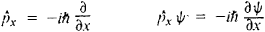It can be shown that the spectrum of its eigenvalues is continuous and that the probability amplitude ‹ x|p › is a de Broglie wave (>|p › is the eigenvector of the momentum operator p). If the energy of a system is given as a function of the coordinates and momentums of the particles H(p, x), then knowledge of the commutator [x, p] is sufficient to find [̂H, ̂p] and [̂H, ̂x], as well as tjhe energy levels as eigenvalues of the operator of total energy ̂H.

From the definition of the angular momentum ̂Mz = xpyyPx, … it can be found that [̂Mx, ̂My] = iℏMz. These commutative relations are also valid when the spins of the particles are taken into consideration. They prove sufficient to determine the eigenvalue of the square of the total angular momentum, ̂M2 = ℏ2j(j + 1), where the quantum number j is an integer or half-integer, and its components Mz = mℏ for m = -j, -j + 1, …, +j.

The equations of motion of a quantum-mechanical system can be written in two forms: in the form of an equation for the state vector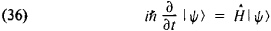—Schrödinger’s form of the equation of motion—and in the form of an equation for the operators (q-numbers)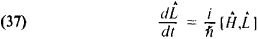—Heisenberg’s form of the equations of motion, which is closest to classical mechanics. In particular, it follows from Heisenberg’s form that the average values of physical quantities change according to the laws of classical mechanics; this proposition is called the Ehrenfest theorem.

The presence of two components that are totally different in nature is characteristic of the logical structure of quantum mechanics. The state vector (the wave function) is uniquely defined at any moment in time if it is defined at the initial moment. In this respect the theory is totally deterministic. But the state vector is not an observable quantity. Only statistical (probabilistic) predictions can be made concerning quantities that are observable on the basis of knowledge of |Ψ›. The results of individual measurement of a quantum entity are, strictly speaking, unpredictable in the general case. Attempts have been made to revive the idea of total determinism in the classical sense by introducing the assumption that the quantum-mechanical description is incomplete. For example, the hypothesis has been advanced that quantum entities have additional degrees of freedom—“latent parameters”—consideration of which would make the behavior of a system totally deterministic in the sense of classical mechanics; uncertainty arises only because these latent parameters are unknown and are not taken into account. However, J. von Neumann proved the theorem that it is impossible to give a nonstatistical interpretation of quantum mechanics while retaining its principal assumption on the correspondence between observables (physical quantities) and operators.

### REFERENCES

#### Classical works

Heisenberg, W. Fizicheskie printsipy kvantovoi teorii. Leningrad-Moscow, 1932.
Dirac, P. Printsipy kvantovoi mekhaniki. Moscow, 1960. (Translated from English.)
Pauli, W. Obshchie printsipy volnovoi mekhaniki. Moscow-Leningrad, 1947. (Translated from German.)
Neumann, J. von. Matematicheskie osnovy kvantovoi mekhaniki. Moscow, 1964. (Translated from German.)

#### Textbooks

Landau, L. D., and E. M. Lifshits. Kvantovaia mekhanika, 2nd ed. Moscow, 1963. (Teoreticheskaia fizika, vol. 3.)
Blokhintsev, D. I. Osnovy kvantovoi mekhaniki, 4th ed. Moscow, 1963.
Davydov, A. S. Kvantovaia mekhanika. Moscow, 1963.
Sokolov, A. A., Iu. M. Loskutov and I. M. Ternov. Kvantovaia me khanika. Moscow, 1962.
Bohm, D. Kvantovaia teoriia. Moscow, 1961. (Translated from English.)
Feynman, R., et al. Feinmanovskie lektsiipo fizike, fascs. 8 and 9. Moscow, 1966-67. (Translated from English.)
Schiff, L. Kvantovaia mekhanika, 2nd ed. Moscow, 1959. (Translated from English.)
Fermi, E. Kvantovaia mekhanika. Moscow, 1965. (Translated from English.)

#### Popular books

Born, M. Atomnaia fizika, 3rd ed. Moscow, 1970. (Translated from English.)
Peierls, R. E. Zakony prirody, 2nd ed. Moscow, 1962. (Translated from English.)

V. B. BERESTETSKII

## quantum mechanics

[′kwän·təm mi′kan·iks]
(physics)
The modern theory of matter, of electromagnetic radiation, and of the interaction between matter and radiation; it differs from classical physics, which it generalizes and supersedes, mainly in the realm of atomic and subatomic phenomena. Also known as quantum theory.
McGraw-Hill Dictionary of Scientific & Technical Terms, 6E, Copyright © 2003 by The McGraw-Hill Companies, Inc.

## quantum mechanics

The branch of physics developed in the first part of the 20th century that was highly successful in explaining the behavior of atoms, molecules and nuclei. Developed between 1900 and 1930 and combined with Einstein's general and special theory of relativity, it revolutionized the field of physics. The new concepts, which were the particle properties of radiation, the wave properties of matter, quantization of physical properties and the idea that one can no longer know exactly where a single particle such as an electron is at any one instance were necessary to explain all of the new experimental evidence that was available at the time. For example, quantum mechanics explains the behavior of semiconductors which are used to make the myriad devices we use every day. See wave-particle duality, quantum state and quantum computing.

Following are the important contributors to the foundation of quantum mechanics and the principles they uncovered.

Year  Researcher    Contribution

1905  Einstein      Photoelectric effect

1913  Bohr          Spectra theory

1922  Compton       Photon scattering

1924  Pauli         Exclusion principle

1925  de Broglie    Matter waves

1926  Schroedinger  Wave equation

1927  Heisenberg    Uncertainty principle

1927  Davison &     Wave properties of
Germer        electrons

1927  Born          Interpretation of the
wavefunction

Copyright © 1981-2019 by The Computer Language Company Inc. All Rights reserved. THIS DEFINITION IS FOR PERSONAL USE ONLY. All other reproduction is strictly prohibited without permission from the publisher.
References in periodicals archive ?
How hard is it to reconcile philosophy with quantum mechanics?
"Using our quantum radio, we want to try to listen to and control the quantum vibrations of heavy objects, and explore experimentally what happens when you mix quantum mechanics and gravity," Gely said.
In addition, the basic deficiency of hidden-variable nonexistence proofs is that they are derived within the context of quantum mechanics. By its very nature, quantum mechanics is a probabilistic theory--so it is not surprising that such "proofs" find that deterministic results cannot be derived from quantum mechanics.
The project of Lewis's book is stated on the first page: "If the theory of quantum mechanics by itself does not tell us how to conceive of the world behind quantum phenomena, then it is up to us to construct such a conception." Surely, after twenty-five centuries of questioning and honing the tools for investigating being and the nature of reality, metaphysicians ought to be eager to take up the challenge.
In this formalism of quantum mechanics, commutation relation between coordinate and its momentum should be deformed in form of
While Newtonian physics was deterministic, essentially precluding free-will, quantum mechanics may allow for free-will.
With so many mechanical explanations for quantum mechanical behaviors provided by the walker experiments, it becomes necessary to examine any behaviors of quantum mechanics which are not described by these experiments.
Rich in math and science, Quantum Mechanics is highly recommended for any college, professor or student pursuing an undergraduate course of study in quantum mechanics.
Table 1: Similarities between Theory of Relativity and Quantum Mechanics Both are considered the cornerstones of modern physics.
For advanced undergraduate science, math, and engineering students who have taken introductory courses in linear algebra, calculus, and classical and quantum mechanics, as well as graduate students and scientists and engineers, Blumel (Wesleyan U.) introduces quantum chaos and semiclassical physics.
Only with an understanding of quantum mechanics can the basic nature of all things, from the most massive black hole to the tiniest subatomic particle, be truly understood.
SUMMARY: This article sets forth and discusses the Ithaca Interpretation of Quantum Mechanics (IIQM).

Site: Follow: Share:
Open / Close